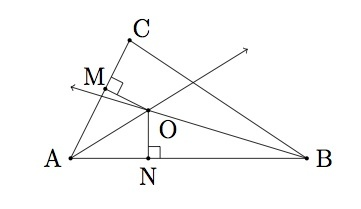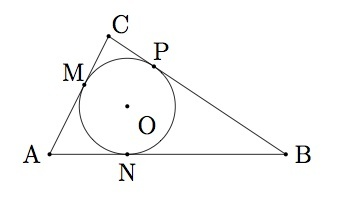# Inscribing a circle in a triangle I

Alignments to Content Standards: G-C.A.3

The goal of this task is to show how to draw a circle which is tangent to all three sides of a given triangle: that is, the circle touches each side of the triangle in a single point. Suppose $ABC$ is a triangle as pictured below with ray $\overrightarrow{AO}$ the bisector of angle $A$ and ray $\overrightarrow{BO}$ the bisector of angle $B$:Also pictured are the point $M$ on $\overline{AC}$ so that $\overline{OM}$ meets $\overline{AC}$ in a right angle and similarly point $N$ on $\overline{AB}$ is chosen so that $\overline{ON}$ meets $\overline{AB}$ in a right angle.

1. Show that triangle $AOM$ is congruent to triangle $AON$.
2. Show that $\overline{OM}$ is congruent to segment $\overline{ON}$.
3. Arguing as in parts (a) and (b) show that if $P$ is the point on $\overline{BC}$ so that $\overline{OP}$ meets $\overline{BC}$ in a right angle then $OP$ is also congruent to $\overline{OM}$.
4. Show that the circle with center $O$ and radius $|OM|$ is inscribed inside triangle $ABC$.

## IM Commentary

This task shows how to inscribe a circle in a triangle using angle bisectors. A companion task, ''Inscribing a circle in a triangle II'' stresses the auxiliary remarkable fact that comes out of this task, namely that the three angle bisectors of triangle $ABC$ all meet in the point $O$. In order to complete part (d) of this task, students need to know that the tangent line to a circle at a point $p$ is characterized by being perpendicular to the radius of the circle at $p$.

This task is primarily intended for instruction purposes but parts (a) and (b) could be used for assessment. The teacher is encouraged to draw many different triangles both to provide a broader context for the problem and to see where the inscribed circle lies depending on the shape of the triangle. If students no how to bisect an angle and draw the segment from a point to a line, meeting the line in a right angle, then they could perform the entire construction with straightedge and compass. This particular construction has a great reward at the end, namely being able to produce the inscribed circle inside the triangle.

## Solution

1. Since ray $\overrightarrow{AO}$ bisects angle $A$ this means that angle $MAO$ is congruent to angle $NAO$. We also know that angles $AMO$ and $ANO$ are both right angles and so they are congruent. Since

\begin{align} m(\angle MOA) &= 180 - m(\angle MAO) - m(\angle AMO) \\ m(\angle NOA) &= 180 - m(\angle NAO) - m(\angle ANO) \end{align}

Since $m(\angle MAO) = m(\angle NAO)$ and $m(\angle AMO) = m(\angle ANO)$ we conclude that $m(\angle (MOA) = m(\angle NOA)$. Triangles $MAO$ and $NAO$ share side $\overline{AO}$ and so by ASA they are congruent.

Alternatively, triangles $MAO$ and $NAO$ are right triangles. Their hypotenuses are congruent and they share the congruent angles $MAO$ and $NAO$. If students are familiar with the Hypothenuse Angle congruent theorem, this is sufficient information to conclude that triangles $MAO$ and $NAO$ are congruent.

2. Segments $\overline{OM}$ and $\overline{ON}$ are corresponding sides of congruent triangles $MAO$ and $NAO$, by part (a), and so they must be congruent.
3. We will use a new picture with ray $\overrightarrow{BO}$ replaced by ray $\overrightarrow{CO}$The argument from part (a) can be produced word for word with the point $P$ replacing $N$. Alternatively, looking at the argument for parts (a) and (b) they used the facts that $ABC$ is a triangle, that $\overrightarrow{AO}$ bisects angle $A$ and that $\overrightarrow{BO}$ bisects angle $B$. Since the vertices of triangle $ABC$ can be labelled in any way we choose, we could switch the labels for $B$ and $C$ and then part (a) would show that triangles $AOM$ and $AOP$ are congruent and part (b) would conclude that $\overline{OP}$ is congruent to $\overline{OM}$.

As in part (a), students can also use the Hypotenuse Angle congruence theorem to show that triangles $COM$ and $COP$ are congruent ($\overline{CO}$ is congruent to $\overline{CO}$ and angle $MCO$ is congruent to angle $PCO$). It then follows that segments $\overline{OM}$ and $\overline{OP}$ are congruent as they are corresponding parts of congruent triangles.

4. The circle with center $O$ and radius $|OM|$ meets $\overline{AB}$ at $N$, $\overline{AC}$ at $M$, and $\overline{BC}$ at $P$. Moreover, the radii of the circle at these three points are perpendicular to the segments. This means that the lines $\overleftrightarrow{AC}$, $\overleftrightarrow{AB}$, and $\overleftrightarrow{BC}$ are all tangent lines for the circle. So the circle radius $|OM|$ and center $O$ is inscribed in triangle $ABC$ as pictured below: